# Solutions to SQA examination

## 2004

```Section A
1. C		11. B
2. D		12. E
3. D		13. E
4. C		14. C
5. B		15. E	SQA say D?
6. B		16. D
7. A		17. A
8. A		18. D
9. E		19. B
10.E		20. C

Section B

21.a.	Speed is a scalar with magnitude only.
Velocity is a vector with magnitude and velocity.

b.i.Distance(1) = 	Speed x time
10.0km/h x 0.5h
5km

Distance(2) = 	8.0km/h x 1.5
12km

Distance(Total) = Distance(1)+Distance(2)
Distance(Total) = 5km + 12km
Distance(Total) = 17km

b.ii.Draw an accurate scale diagram and take careful measurements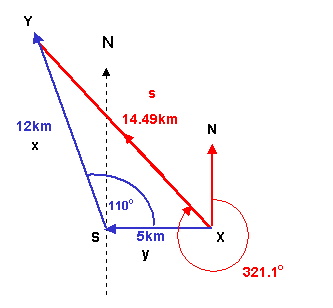or use trigonometry.

Use the cosine rule to calculate the resultant displacement.

s2=x2 + y2 -2xycosX
s2=122 + 52 -2x12x5cos110o
s2=169 + 41.04
s = SQRT(210.4)
s =14.49km

Use the sine rule to calculate angle X and hence the bearing.

s/sinS = x/sinX
sinX = (xsinS)/s
sinX = (12sin110o)/14.49
sinX = (11.28)/14.49
sinX = 0.778

X = 51.1o

bearing 321.1o

b.iii.velocity = displacement/time

s = 14.49km		v = 14.49/2
t = 2 h			v = 7.24km/h

c.	Time for Leeuvin to sail directly:

t = s/v
t = 14.49/7.5
t = 1.932h = 1hour 55.9minutes

Time for Leeuvin to reach Y  after the Mir passes X
1h 55.9min + 15min = 2h 10.9min

This is longer than the 2h journey of the Mir.

Leeuvin reaches Y 10.9 minutes after Mir.

22.a.i.u = 60m/s	v2 = u2 + 2as
v = 0m/s	s = (v2 - u2)/2a
t = 40s
s = ? 		a = (v-u)/t
a = (0 - 60)/40 = -1.5m/s/s

s = (02 - 602)/2x-1.5
s = -3600/-3
s = 1200m

a.ii.Favg = m(v-u)/t

m = 7.5x105kg			Favg = 7.5x105(0-60)/40
u = 60m/s			Favg = -11.25x105N
v = 0m/s			The force acts in the opposite direction
t = 40s 			to the motion.

Kinetic energy equated to the work done by the braking
force also produces the above answer.

b.	Irms = 2.5x103A			Prms  = Irms x Vrms
P = 8.5x106(J/s) or (W)		Vrms = Prms/Irms
Vrms = 8.5x106/2.5x103
Vrms = 3400V

23.a.	As the manifold is stationary the upward forces
balance the downward forces. Tension = Weight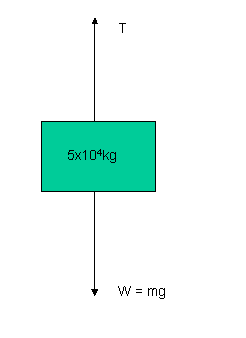w = mg
w = 5.0x104kg x 9.8N/kg
w = 490000N

T = 490000N (upwards)

b.i.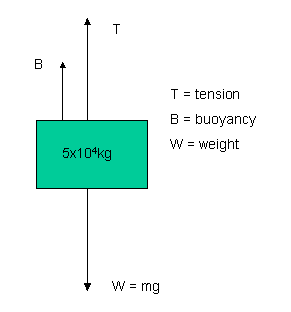b.ii.Again as the manifold is stationary the upward forces
balance the downward forces.

T + B = W
2.5x105 + B = 490000N
B = 490000 - 250000
B = 240000N

The buoyancy force is a result of the pressure
difference between the upper and lower surfaces.

B = (Plower-Pupper)A
DP = B/A
DP = 240000N/8.0m2
DP = 30000Pa	As required

c.	There is no change in the pressure difference.

Pliquid(top) = rgh
Pliquid(bottom) = rg(h+Dh)

DP = Pliquid(bottom) - Pliquid(top)
DP = rgDh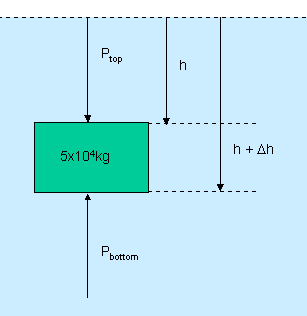Only the difference in the depth(Dh) of the top and
bottom surfaces affects the pressure difference.

24.a.i.r = 2.0W
emf = 9.0V (open circuit voltage)
Vtpd = 7.8V

Vlost = emf - Vtpd
Vlost = 9.0 - 7.8
Vlost = 1.2V

Vlost = Ir
I = Vlost/r
I = 1.2/2.0
I = 0.6A

Vtpd = VR
VR = IR
R = VR/I
R = 7.8/0.6
R = 13W

24.a.ii.When S1 is closed there is a current through the internal
resistor. The voltage drop across this resistor is "lost"
and produces the decreased reading on the voltmeter.

b.	The 30W resistor in parallel with the original
load resistor decreases the effective resistance
of the load. More current therefore flows through
the internal resistor resulting in more "lost volts".
This means the voltmeter reading decreases.

25.a.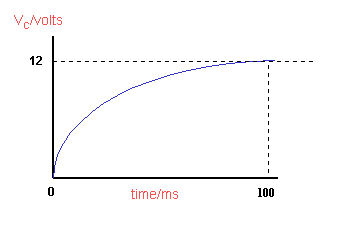When fully charged the current in the circuit falls to
zero and the voltage across the capacitor is equal to the
supply voltage.

25.bi.	I = 20mA
R = 400W
VR = IR

VR = 20x10-3x400
VR = 8V

Vsupply = VR + VC
VC = Vsupply - VR
VC = 12 - 8
VC = 4V

b.ii.	E = 1/2(CV2)
E = 0.5 x 100x10-6 x 42
E = 0.0008J (800mJ)

c.	Reduce the value of the resistor in the circuit
to less than 400W.

d.	The charging time is less. This means the capacitor
must have a value less than 400mF

26.a.	Differential op-amp
Vout = (Rf/R1)(V2-V1)
Vout = (120/10)(7.52-7.50)
Vout = 12 x 0.02
Vout = 0.24V

b.	As the temperature increases the:

Resistance of the thermistor decreases increasing V2 and Vout.
When Vout increases to 0.7V or above the transistor conducts.
The electromagnet in the relay gets magnitised and closes
the switch to the alarm.

c.	Vout = (Rf/R1)(V2-V1)
0.72 = 12(V2 - 7.50)
V2 = (0.72/12) + 7.50
V2 = 0.06 + 7.50
V2 = 7.56V

This corresponds to a temperature of 36oC

27.a.i.Angle air = 82o
Angle liquid = 45o

nliquid(red) = sin(air)/sin(liquid)
nliqiud(red) = sin82o/sin45o
nliquid(red) = 0.990/0.707
nliquid(red) = 1.40

a.ii.The angle of refraction for blue light is greater.

sinqair = nsinqliquid
qair = sin-1(nsinqliquid)
As nliquid(blue) > liquid(red) qair must be greater.

Note: qair is the angle of refraction.

b.	qcritical = sin-1(1/n)
qcritical = sin-1(1/1.44)
qcritical = sin-1(0.694)
qcritical = 43.9o

This means that light incident at 45o will be totally
internally reflected.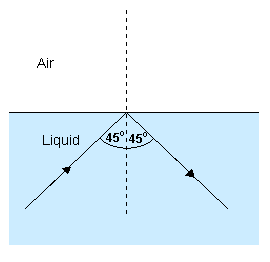28.a.i.A passing photon can encourage or stimulate an electron
to fall from a higher energy level in an atom to a lower
one. This will happen if the passing photon has the same
energy as the energy gap between the two energy levels
in the atom.

a.ii.Amplification is produced because each photon produced by
stimulated emission becomes a new stimulating photon. One
becomes two, two becomes four, four becomes eight and so on.

b.	Grating Equation :	dsinq = nl

d = ?m
n = 1
q = 37/2o =18.5o
l = 633nm = 633 x10-9m

d = nl/sinq
d = 1 x 633 x10-9/sin18.5o
d = 1.995x10-6m

Lines per metre = 1/d
Lines per metre = 1/1.995x10-6
Lines per metre = 501271

c.	The wavelength has been decreased. Shorter wavelengths
are diffracted less than longer ones so the maxima are
closer together.

29.a.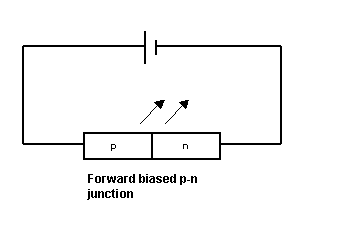b.	In forward bias the junction conducts if the applied
voltage is high enough. Higher energy electrons flowing
from the n-type material fall into holes, at a lower energy
level, in the p-type material. The electrons give up energy
in the form of photons as they do this.

c.i.	E = 3.68x10-19J			E = hf
h = 6.63x10-34Js			f = E/h
f = ?				f = 3.68x10-19/6.63x10-34
f = 5.55x1014Hz

l = v/f
l = 3x108/5.55x1014
l = 540nm

ii.	E = 3.68x10-19J			E = qV
q = 1.60x10-19C			V = E/q
V = 				V = 3.68x10-19/1.60x10-19
V = 2.3V

30.a.i.	92 is the atomic number. The number of protons
in the uranium nucleus.

a.ii.235 is the mass number. The number of protons
plus neutrons in the uranium nucleus.

b.	The two neutrons produced in the fission reaction
can be absorbed by two other uranium nuclei and
produce two more fissions.

c.	Total mass before = (390.173 + 1.675)x10-27
=  391.848x10-27kg

Total mass after = (232.242 + 155.884 + 2x1.675)x10-27
391.476x10-27kg

Mass defect(Dm) = (391.848 - 391.476)x10-27
= 0.372x10-27kg

E = Dmc2
E = 0.372x10-27 x (3x108)2
E = 33.48x10-12J

END OF QUESTION PAPER